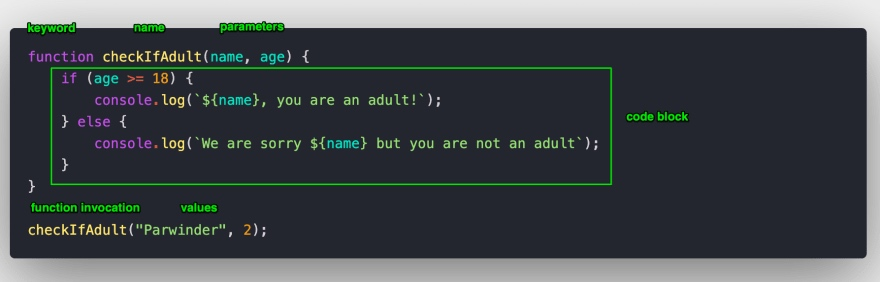# 10 – JavaScript 中的函数### 什么是函数？它在 JavaScript 中扮演什么角色？

1. 使用 function 关键字声明；
2. 函数名字紧跟其后，它就是被调用时使用的名字。
3. 你可以给函数传递参数，那些值可以是动态的。
4. 形参传递给函数的实参。
5. 当函数被调用时，代码块将会被执行。
6. 代码块是被中括号包裹的。### 函数返回值

``````function sum(a, b) {
return a + b; // You return the value using return keyword
}

const x = sum(2,5);
const y = sum(7,9);
console.log(x); // 7
console.log(y); // 16
``````

``````console.log("Hello World!");
// Hello World
// undefined
``````

### JavaScript 自带的函数

``````console.log(parseInt()); // NaN (Not a number)
console.log(parseInt("234xyz")); // 234, parseInt ignore non integer
console.log(Math.random()); // 0.00746544513267
console.log(Date.now()); // 1590557812411
``````

## JavaScript 中函数的类型

### 函数声明

``````function greet(firstName = "new", lastName = "user") {
return `Hello \${firstName} \${lastName}`;
}

console.log(greet("Parwinder", "Bhagat")); // Hello Parwinder Bhagat
console.log(greet()); // Hello new user
``````

greet 函数就是函数声明的例子。

### 函数表达式

``````const greet = function (firstName = "new", lastName = "user") {
return `Hello \${firstName} \${lastName}`;
}

console.log(greet("Parwinder", "Bhagat")); // Hello Parwinder Bhagat
console.log(greet()); // Hello new user
``````

### IIFE (立即执行函数)

IIFE 是一个声明和执行同时发生的函数。通过匿名函数和小括号来创建，然后通过在其后增加一个小括号调用。

``````(function(name){ // function expression enclosed in ()
console.log(`Hello \${name}`); // Hello Parwinder
})("Parwinder"); // Immediately called by using () in the end. Yes we can pass arguments
``````

### 箭头函数

``````const hello = () => {
return "Hello World!";
}

console.log(hello()); // Hello World
``````

``````const hello = () => "Hello World!";
console.log(hello()); // Hello World
``````

``````const hello = (name) => `Hello \${name}`;
console.log(hello("Parwinder")); // Hello Parwinder
``````

``````const hello = name => `Hello \${name}`;
console.log(hello("Parwinder")); // Hello Parwinder
``````